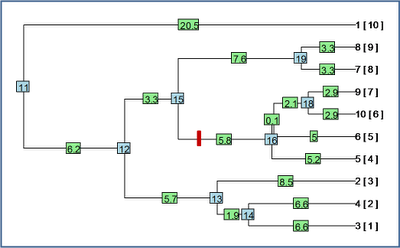## Monday, June 13, 2011

### Re-rooting along a terminal edge

Ok, my wrapper function, reroot(), also now can re-root the tree along terminal edges. The way I did this was by re-rooting the tree at the parent node of the target edge; dropping the target tip; then creating a new two taxon "phylo" object with the target edge split between an edge leading to the target tip and the new root edge for the remainder of the tree. Finally, I used bind.tree() to reattach the remaining tree to the two taxon "phylo" object.

The code is simple enough, so I have a reduced version (without error handling, etc.) below. I will post a completed version shortly to my R-phylogenetics page.

Code below:

reroot<-function(tree,node.number,position){
if(node.number>length(tree\$tip)){
# first, re-root the tree at node.number
tr<-root(tree,node=node.number,resolve.root=T)
# now re-allocate branch length to the two edges descending
# from the new root node
b<-sum(tr\$edge.length[tr\$edge==(length(tree\$tip)+1)])
tr\$edge.length[tr\$edge==(length(tree\$tip)+1)]<-c(position, b-position)
} else {
# first, root the tree at the parent of node.number
tr1<-root(tree,node=tree\$edge[match(node.number, tree\$edge[,2]),1])
# now drop tip
tr1<-drop.tip(tr1,tree\$tip.label[node.number])
# create phylo object
tr2<-list(edge=matrix(c(3L,1L,3L,2L),2,2,byrow=T), tip.label=c(tree\$tip.label[node.number],"NA"), edge.length=c(tree\$edge.length[match(node.number,tree\$edge[,2])] -position,position),Nnode=1)
class(tr2)<-"phylo"
tr<-bind.tree(tr2,tr1,where=which(tr2\$tip.label=="NA"))
}
return(tr)
}

Let's try it out. First, a random birth-death tree:Here, the blue numbers are internal node numbers; the green numbers are edge lengths; and the numbers in [ square parentheses ] after each tip label are the node numbers for the tips.

First, let's try and re-root this tree 1.0 units of edge length along the branch leading to node #16 (marked with a red hashmark on the figure above):

> test1<-reroot(tree,node.number=16,position=1.0)
> plot(test1)Cool - it seems to work.

Now, let's try re-rooting the tree along a terminal edge - say the edge leading to taxon "2". We'll do this 8.0 units along this edge (which is ~8.5 units long in total). We can actually do this without knowing the node number for taxon "2" as follows:

> test2<-reroot(tree,node.number=which(tree\$tip.label=="2"), position=8.0)
> plot(test2)And we're done.

I just wrote this simple function this morning, but it is for a project I am presently working on, so if you find any bugs - I would be happy to hear about it.

#### 1 comment:

1.Nice! I tried it on a several trees and it appears to work well.

Note: due to the very large amount of spam, all comments are now automatically submitted for moderation.